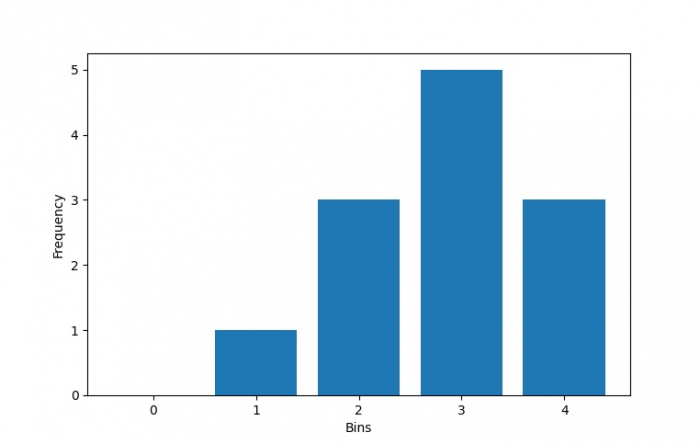# How to compute the histogram of a tensor in PyTorch?

The histogram of a tensor is computed using torch.histc(). It returns a histogram represented as a tensor. It takes four parameters: input, bins, min and max. It sorts the elements into equal width bins between min and max. It ignores the elements smaller than the min and greater than the max.

## Steps

• Import the required library. In all the following Python examples, the required Python libraries are torch and Matplotlib. Make sure you have already installed them.

• Create a tensor and print it.

• Compute torch.histc(input, bins=100, min=0, max=100). It returns a tensor of histogram values. Set bins, min, and max to appropriate values according to your need.

• Print the above calculated histogram.

• Visualize the histogram as a bar diagram.

## Example 1

# Python program to calculate histogram of a tensor
# import necessary libraries
import torch
import matplotlib.pyplot as plt

# Create a tensor
T = torch.Tensor([2,3,1,2,3,4,3,2,3,4,3,4])
print("Original Tensor T:\n",T)

# Calculate the histogram of the above created tensor
hist = torch.histc(T, bins = 5, min = 0, max = 4)
print("Histogram of T:\n", hist)

## Output

Original Tensor T:
tensor([2., 3., 1., 2., 3., 4., 3., 2., 3., 4., 3., 4.])
Histogram of T:
tensor([0., 1., 3., 5., 3.])

## Example 2

# Python program to calculate histogram of a tensor
# import necessary libraries
import torch
import matplotlib.pyplot as plt

# Create a tensor
T = torch.Tensor([2,3,1,2,3,4,3,2,3,4,3,4])
print("Original Tensor T:\n",T)

# Calculate the histogram of the above created tensor
hist = torch.histc(T, bins = 5, min = 0, max = 4)

# Visualize above calculated histogram as bar diagram
bins = 5
x = range(bins)
plt.bar(x, hist, align='center')
plt.xlabel('Bins')
plt.ylabel('Frequency')
plt.show()

## Output

Original Tensor T:
tensor([2., 3., 1., 2., 3., 4., 3., 2., 3., 4., 3., 4.])